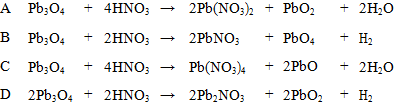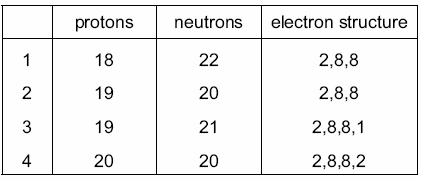FORM 1 CHEMISTRY

ONLINE TEST SERIES

SEPARATION OF MIXTURES

1.What is the formula for the compound formed by calcium and nitrogen?

2.The  oxide  Pb3O4 reacts with dilute nitric acid  to form lead(II)  nitrate,  lead(IV)  oxide  and  another product.What is the equation for this reaction?3.A certain sample of element Z contains 60% of 69Z and 40% of 71Z. What is the relative atomic mass of element Z in this sample?

4.The table shows the numbers of particles present in the nuclei of four atoms or ionsWhich two particles belong to the same element?

5.What is the electronic structure of an atom with a proton number 5 and a nucleon number 11?

6.An element S has the proton number 18. The next element in the Periodic Table is an element T.Which statement is correct?

7.Which arrangement of electrons is that of a gas normally used to fill light bulbs?

8.The reaction of ethanal and oxygen can be represented by the unbalanced equation below.

__ CH3CHO + __ O2 → __ CO2 + __ H2O . When the equation is balanced using the smallest possible integers, what is the coefficient for O2?

9. Copper can react with nitric acid as follows.

3Cu +_HNO3 ® _Cu(NO3)2 +_H2O + _NO . What is the coefficient for HNO3  when the equation is balanced?

10.A certain sample of element Z contains 60% of 69Z and 40% of 71Z. What is the relative atomic mass of element Z in this sample?

Question 1 of 10